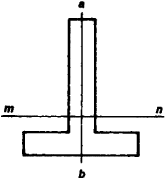Neutral Axis

(redirected from neutral axes)
Also found in: Dictionary.

neutral axis

[′nü·trəl ′ak·səs]
(mechanics)
In a beam bent downward, the line of zero stress below which all fibers are in tension and above which they are in compression.

Axis, Neutral

(in strength of materials), for a beam that undergoes bending, a line in a cross section of the beam such that at all points of the line the normal stresses parallel to the axis of the beam are equal to zero. The neutral axis divides the cross section into two parts: the normal stresses are tensile in one part and compressive in the other. If ab (see Figure 1)—the line through which the plane of action of the external forces passes—is one of the principal axes of inertia for the cross section shown in Figure 1, then the neutral axis mn will be the second principal axis. The locus of all the neutral axes forms the neutral surface of a beam.Figure 1

neutral axis

An imaginary line in a beam, shaft, or other member, subjected to bending, where there is no tension or compression and where no deformation takes place.
References in periodicals archive ?
When assembled into the piston, the top two rings are closed from their free shape and the change of curvature and torsion of their neutral axes causes internal stress.
The model regards eccentricities in cross-bracings to bottom flange connections, different levels of neutral axes of truss bottom flange members and level of supports as well as the fact that live load is applied to girders at connections of cross beams.
Thus, the task of this work is to derive a theoretic solution for very important problem and in many cases the most complicated one--calculation of neutral axes location by ZI method for cross-section of flexural members when strain of any layer is not known beforehand together with N [not equal to] 0 or/and P [not equal to] 0.

Site: Follow: Share:
Open / Close